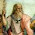## Thursday, December 09, 2010

### MuonThe Moon's cosmic ray shadow, as seen in secondary muons generated by cosmic rays in the atmosphere, and detected 700 meters below ground, at the Soudan II detector. Composition: Elementary particle Particle statistics: Fermionic Group: Lepton Generation: Second Interaction: Gravity, Electromagnetic, Weak Symbol(s): μ− Antiparticle: Antimuon (μ+) Theorized: — Discovered: Carl D. Anderson (1936) Mass: 105.65836668(38) MeV/c2 Mean lifetime: 2.197034(21)×10−6 s Electric charge: −1 e Color charge: None Spin: 1⁄2

The muon (from the Greek letter mu (μ) used to represent it) is an elementary particle similar to the electron, with a negative electric charge and a spin of ½. Together with the electron, the tau, and the three neutrinos, it is classified as a lepton. It is an unstable subatomic particle with the second longest mean lifetime (2.2 µs), exceeded only by that of the free neutron (~15 minutes). Like all elementary particles, the muon has a corresponding antiparticle of opposite charge but equal mass and spin: the antimuon (also called a positive muon). Muons are denoted by μ and antimuons by μ+. Muons were previously called mu mesons, but are not classified as mesons by modern particle physicists (see History).

Muons have a mass of 105.7 MeV/c2, which is about 200 times the mass of an electron. Since the muon's interactions are very similar to those of the electron, a muon can be thought of as a much heavier version of the electron. Due to their greater mass, muons are not as sharply accelerated when they encounter electromagnetic fields, and do not emit as much bremsstrahlung radiation. Thus muons of a given energy penetrate matter far more deeply than electrons, since the deceleration of electrons and muons is primarily due to energy loss by this mechanism. So-called "secondary muons", generated by cosmic rays hitting the atmosphere, can penetrate to the Earth's surface and into deep mines.

As with the case of the other charged leptons, the muon has an associated muon neutrino. Muon neutrinos are denoted by νμ.

## History

Muons were discovered by Carl D. Anderson and Seth Neddermeyer at Caltech in 1936, while studying cosmic radiation. Anderson had noticed particles that curved differently from electrons and other known particles when passed through a magnetic field. They were negatively charged but curved less sharply than electrons, but more sharply than protons, for particles of the same velocity. It was assumed that the magnitude of their negative electric charge was equal to that of the electron, and so to account for the difference in curvature, it was supposed that their mass was greater than an electron but smaller than a proton. Thus Anderson initially called the new particle a mesotron, adopting the prefix meso- from the Greek word for "mid-". Shortly thereafter, additional particles of intermediate mass were discovered, and the more general term meson was adopted to refer to any such particle. To differentiate between different types of mesons, the mesotron was in 1947 renamed the mu meson (the Greek letter μ (mu) corresponds to m).
It was soon found that the mu meson significantly differed from other mesons: for example, its decay products included a neutrino and an antineutrino, rather than just one or the other, as was observed with other mesons. Other mesons were eventually understood to be hadrons—that is, particles made of quarks—and thus subject to the residual strong force. In the quark model, a meson is composed of exactly two quarks (a quark and antiquark) unlike baryons, which are composed of three quarks. Mu mesons, however, were found to be fundamental particles (leptons) like electrons, with no quark structure. Thus, mu mesons were not mesons at all (in the new sense and use of the term meson), and so the term mu meson was abandoned, and replaced with the modern term muon.

Another particle (the pion, with which the muon was initially confused) had been predicted by theorist Hideki Yukawa:

"It seems natural to modify the theory of Heisenberg and Fermi in the following way. The transition of a heavy particle from neutron state to proton state is not always accompanied by the mission of light particles. The transition is sometimes taken up by another heavy particle."

The existence of the muon was confirmed in 1937 by J. C. Street and E. C. Stevenson's cloud chamber experiment. The discovery of the muon seemed so incongruous and surprising at the time that Nobel laureate I. I. Rabi famously quipped, "Who ordered that?"

In a 1941 experiment on Mount Washington in New Hampshire, muons were used to observe the time dilation predicted by special relativity for the first time.

## Muon sources

Since the production of muons requires an available center of momentum frame energy of 105.7 MeV, neither ordinary radioactive decay events nor nuclear fission and fusion events (such as those occurring in nuclear reactors and nuclear weapons) are energetic enough to produce muons. Only nuclear fission produces single-nuclear-event energies in this range, but do not produce muons as the production of a single muon would violate the conservation of quantum numbers (see under "muon decay" below).

On Earth, most naturally occurring muons are created by cosmic rays, which consist mostly of protons, many arriving from deep space at very high energy

About 10,000 muons reach every square meter of the earth's surface a minute; these charged particles form as by-products of cosmic rays colliding with molecules in the upper atmosphere. Travelling at relativistic speeds, muons can penetrate tens of meters into rocks and other matter before attenuating as a result of absorption or deflection by other atoms.

When a cosmic ray proton impacts atomic nuclei of air atoms in the upper atmosphere, pions are created. These decay within a relatively short distance (meters) into muons (the pion's preferred decay product), and neutrinos. The muons from these high energy cosmic rays generally continue in about the same direction as the original proton, at a very high velocity. Although their lifetime without relativistic effects would allow a half-survival distance of only about 0.66 km (660 meters) at most (as seen from Earth) the time dilation effect of special relativity (from the viewpoint of the Earth) allows cosmic ray secondary muons to survive the flight to the Earth's surface, since in the Earth frame, the muons have a longer half-life due to their velocity. From the viewpoint (inertial frame) of the muon, on the other hand, it is the length contraction effect of special relativity which allows this penetration, since in the muon frame, its lifetime is unaffected, but the distance through the atmosphere and earth appears far shorter than these distances in the Earth rest-frame. Both are equally valid ways of explaining the fast muon's unusual survival over distances.

Since muons are unusually penetrative of ordinary matter, like neutrinos, they are also detectable deep underground (700 meters at the Soudan II detector) and underwater, where they form a major part of the natural background ionizing radiation. Like cosmic rays, as noted, this secondary muon radiation is also directional.

The same nuclear reaction described above (i.e. hadron-hadron impacts to produce pion beams, which then quickly decay to muon beams over short distances) is used by particle physicists to produce muon beams, such as the beam used for the muon g − 2 experiment.

## Muon decay

Muons are unstable elementary particles and are heavier than electrons and neutrinos but lighter than all other matter particles. They decay via the weak interaction. Because lepton numbers must be conserved, one of the product neutrinos of muon decay must be a muon-type neutrino and the other an electron-type antineutrino (antimuon decay produces the corresponding antiparticles, as detailed below). Because charge must be conserved, one of the products of muon decay is always an electron of the same charge as the muon (a positron if it is a positive muon). Thus all muons decay to at least an electron, and two neutrinos. Sometimes, besides these necessary products, additional other particles that have a net charge and spin of zero (i.e. a pair of photons, or an electron-positron pair), are produced.

The dominant muon decay mode (sometimes called the Michel decay after Louis Michel) is the simplest possible: the muon decays to an electron, an electron-antineutrino, and a muon-neutrino. Antimuons, in mirror fashion, most often decay to the corresponding antiparticles: a positron, an electron-neutrino, and a muon-antineutrino. In formulaic terms, these two decays are: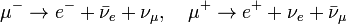$\mu^-\to e^- + \bar\nu_e + \nu_\mu,~~~\mu^+\to e^+ + \nu_e + \bar\nu_\mu$.
The mean lifetime of the (positive) muon is 2.197 019 ± 0.000 021 μs. The equality of the muon and anti-muon lifetimes has been established to better than one part in 104.

The tree-level muon decay width is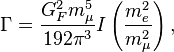$\Gamma=\frac{G_F^2 m_\mu^5}{192\pi^3}I\left(\frac{m_e^2}{m_\mu^2}\right),$
where I(x) = 1 − 8x − 12x2lnx + 8x3x4;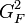$G_F^2$ is the Fermi coupling constant.
The decay distributions of the electron in muon decays have been parameterised using the so-called Michel parameters. The values of these four parameters are predicted unambiguously in the Standard Model of particle physics, thus muon decays represent a good test of the space-time structure of the weak interaction. No deviation from the Standard Model predictions has yet been found.

Certain neutrino-less decay modes are kinematically allowed but forbidden in the Standard Model. Examples forbidden by lepton flavour conservation are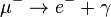$\mu^-\to e^- + \gamma$ and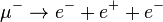$\mu^-\to e^- + e^+ + e^-$.
Observation of such decay modes would constitute clear evidence for physics beyond the Standard Model (BSM). Current experimental upper limits for the branching fractions of such decay modes are in the range 10−11 to 10−12.

## Muonic atoms

The muon was the first elementary particle discovered that does not appear in ordinary atoms. Negative muons can, however, form muonic atoms (also called mu-mesic atoms), by replacing an electron in ordinary atoms. Muonic hydrogen atoms are much smaller than typical hydrogen atoms because the much larger mass of the muon gives it a much smaller ground-state wavefunction than is observed for the electron. In multi-electron atoms, when only one of the electrons is replaced by a muon, the size of the atom continues to be determined by the other electrons, and the atomic size is nearly unchanged. However, in such cases the orbital of the muon continues to be smaller and far closer to the nucleus than the atomic orbitals of the electrons.

A positive muon, when stopped in ordinary matter, can also bind an electron and form an exotic atom known as muonium (Mu) atom, in which the muon acts as the nucleus. The positive muon, in this context, can be considered a pseudo-isotope of hydrogen with one ninth of the mass of the proton. Because the reduced mass of muonium, and hence its Bohr radius, is very close to that of hydrogen[clarification needed], this short-lived "atom" behaves chemically — to a first approximation — like hydrogen, deuterium and tritium.

## Use in measurement of the proton charge radius

The recent culmination of a twelve year experiment investigating the proton's charge radius involved the use of muonic hydrogen. This form of hydrogen is composed of a muon orbiting a proton. The Lamb shift in muonic hydrogen was measured by driving the muon from the from its 2s state up to an excited 2p state using a laser. The frequency of the photon required to induce this transition was revealed to be 50 terahertz which, according to present theories of quantum electrodynamics, yields a value of 0.84184 ± 0.00067 femtometres for the charge radius of the proton.

## Anomalous magnetic dipole moment

The anomalous magnetic dipole moment is the difference between the experimentally observed value of the magnetic dipole moment and the theoretical value predicted by the Dirac equation. The measurement and prediction of this value is very important in the precision tests of QED (quantum electrodynamics). The E821 experiment at Brookhaven National Laboratory (BNL) studied the precession of muon and anti-muon in a constant external magnetic field as they circulated in a confining storage ring. The E821 Experiment reported the following average value (from the July 2007 review by Particle Data Group)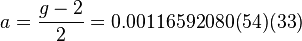$a = \frac{g-2}{2} = 0.00116592080(54)(33)$
where the first errors are statistical and the second systematic.

The difference between the g-factors of the muon and the electron is due to their difference in mass. Because of the muon's larger mass, contributions to the theoretical calculation of its anomalous magnetic dipole moment from Standard Model weak interactions and from contributions involving hadrons are important at the current level of precision, whereas these effects are not important for the electron. The muon's anomalous magnetic dipole moment is also sensitive to contributions from new physics beyond the Standard Model, such as supersymmetry. For this reason, the muon's anomalous magnetic moment is normally used as a probe for new physics beyond the Standard Model rather than as a test of QED (Phys.Lett. B649, 173 (2007)).

1.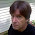I am thinking of Gran Sasso. I hope the people there are safe. :-)

What about muon-muon colliders? From the US Muon -Muon Collider collaboration page:

High energy accelerators and detectors are the light sources and microscopes used to look inside of elementary particles, allowing us to ask deeper questions than ever before: what is the origin of mass?, how did the fundamental particles and forces work together to produce the world around us today?

The majority of contemporary high-energy physics experiments utilize colliders. Colliders are machines that accelerate and guide oppositely directed and tightly focused beams of particles into collision at the intersection point (IP); where enormous amounts of energy are released, creating the conditions, we believe, that occurred during the first moment of our universe.

Operating accelerators generally fall into two classes: hadron accelerators and electron accelerators. In the 50's Tinlot discussed muon storage rings and in the 70's muon colliders were proposed by Skrinsky and Neuffer, but the luminosities were relatively low. In the last two years a renew interest in the idea arose and significant advances in pion capture and cooling has risen the luminosity to levels comparable to those of hadrons or electron machines.

In general terms, the physics that could be studied with a muon-muon collider would be similar to that with an electron-positron machine (Linear Collider). Muons are just heavy electrons (200 times heavier than an electron), but, because of their larger mass, produce much less synchrotron radiation.

We can list at least three advantages for using muons instead of electrons:

Like protons they can be accelerated and stored in circular rings at energies above 250 GeV, as opposed to electrons, which have to be accelerated in linear machines.
Smaller beamstrahlung with the consequent smaller energy loss and narrow energy spread, opens the possibility of more particle per bunch yielding greater luminosity.
Direct s-channel Higgs Higgs boson production is greatly enhanced because the coupling of any Higgs boson is proportional to the mass of the muon.

Smaller radiative correction and associated physics background
No obvious constraints to scaling to multi-TeV energies.
The effective energy in the collision of point particles like muons (or electrons or gammas) is roughly ten times larger than that of protons. Therefore, a circular muon machine would be a factor of 10 smaller than its proton equivalent. A 4-TeV center of mass muon collider, with a 6 km circumference, would have an effective energy, i.e., physics potential for discovery, similar to that of the 80 km Superconducting Super Collider. Also, it would be smaller than a conventional electron linear collider of the same energy; e.g. a 4 TeV electron collider would be 50 km long.
However, there are serious technological challenges to building a muon collider. Firstly, although smaller, there are no guarantees that it would be cheaper. Secondly, it is not completely obvious that the required luminosity L = 1035 cm-2s-1 is achievable.

Of utmost concern are the decays of the muons into electrons and neutrinos. The electrons will have lower energy than the muons and will not be trapped in the ring. They will synchrotron radiate, spreading photons on to the outer wall of the ring, and simultaneously will spiral inward hitting the inner wall of the ring, making a large amount of undesirable radiation. This is a problem for superconducting magnets around the ring. It is also a major concern for the detector, complicating the ability of the physics users to isolate and understand the interesting interactions, such as the production of high mass particles (Higgs bosons?) created at the intersection point.

2.